# How do you type right arrow(→,⇒) in latex?

We use right arrow most in the arrow. Which you can do by default command.

\documentclass{article}
\begin{document}
$A \rightarrow B$
$C \hookrightarrow D$
\end{document}

Output :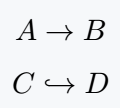But, if you want to make the document beautiful, you need to know the usage of the package.

For example, if you can compare the output above and the output below, then you can understand the difference.

\documentclass{article}
\usepackage{mathabx}
\begin{document}
$A \rightarrow B$
$C \hookrightarrow D$
\end{document}

Output :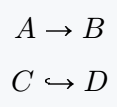## Double right arrow

\xrightarrow and \xRightarrow commands have the same spelling but some differences. And at the same time, two different arrows have been used.

\documentclass{article}
\usepackage{mathabx}
\begin{document}
$A \Rightarrow B$
%Use mathabx package
$E \rightrightarrows F$
\end{document}

Output :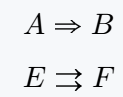## Maps arrow

We rarely see the use of Maps Arrow. However, it is an important symbol used in high-level mathematics.

\documentclass{article}
\usepackage{MnSymbol}
\begin{document}
$(e,f) \mapsto e \bigotimes f$
%Use MnSymbol package
$(e,f) \rightmapsto e \bigotimes f$
\end{document}

Output :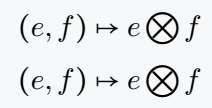## Long right arrow

Each of the above commands will return you a small symbol. So, there are special default commands and packages for a long arrow.

\documentclass{article}
\begin{document}
$2H_2 + O_2 \longrightarrow 2H_2O$
$a_1 \Longrightarrow a_2$
$(e,f) \longmapsto e \bigotimes f$
\end{document}

Output :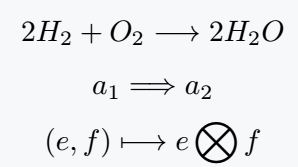MnSymbol package has been used as an optional option below.

\documentclass{article}
\usepackage{MnSymbol}
\begin{document}
$2H_2 + O_2 \longrightarrow 2H_2O$
$S_1 \Longrightarrow S_2$
$(e,f) \longmapsto e \bigotimes f$
\end{document}

Output :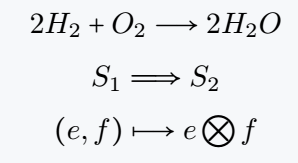## Right circle, curve, and squig arrow

These three arrow symbols are present in the mathabx package. For which \rightsquigarrow, \circlearrowright and \curvearrowbotright command have been called.

\documentclass{article}
\usepackage{mathabx}
\begin{document}
$(x-a)(x-c) = 0 \rightsquigarrow (x-a) = 0$
$\circlearrowright$
$a \curvearrowbotright b$
$c \curvearrowright d$
\end{document}

Output :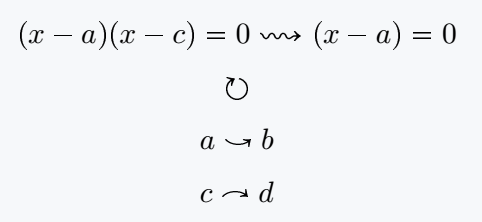## Write text above and below of right arrow

You can add an optional argument to each text over arrow of Mathtools package. Which acts as a denominator. For example

\documentclass{article}
\usepackage{mathtools}
\begin{document}
$\xrightarrow[xyz]{pqr}$
$\xRightarrow[xyz]{pqr}$
$\xmapsto[xyz]{pqr}$
$\xhookrightarrow[xyz]{pqr}$
\end{document}

Output :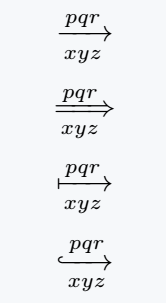## Right harpoon

LaTeX has defined a separate package for the harpoon. However, different packages have discussed this symbol.

\documentclass{article}
\begin{document}
$\rightharpoondown, \rightharpoonup$
$N_2 + 3H_2 \rightleftharpoons 2NH_3$
\end{document}

Output :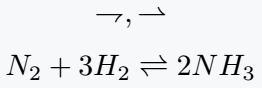#### Md Jidan Mondal

LaTeX expert with over 10 years of experience in document preparation and typesetting. Specializes in creating professional documents, reports, and presentations using LaTeX.